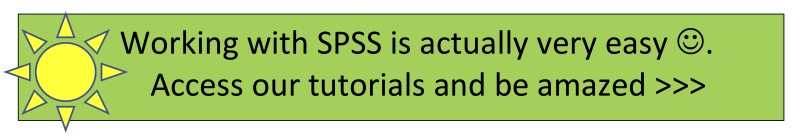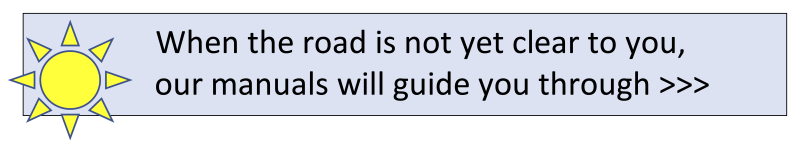Analysing Quantitative data# If the road is not yet clear to you,we will show you the way how to get through.## Quantitative data has to be analysed with quantitative techniques. Although frequencies and crosstabs may be sufficient, a whole battery of statistical analyses is usually performed. Use our five steps method to analyse the data, and read our papers that make statistics much easier to understand.

Quantitative data are data that consist of only numbers. This is not completely true. The datafile might contain text, but text cannot be analysed with the quantitative techniques.

The data are systematically stored in a database. For instance, when a lot of respondents have been questioned, the gathered data are presented as digits. Maybe the first column is text, containing the name of the respondent, but the digits behind every name are representing the answer the person gave to a question.

## To analyse Quantitative data five steps have to be taken

The first stepis always: check your data. If there are wrong scores try to fix it, because finding a mistake later on in the process, the whole procedure might be done all over again. For example, the variable gender can be coded with 0, 1 or 2. You might be surprised the number 2 is in this list. In the codebook you can read that number 1 is be male and 2 is female, so 0 is a wrong number.

A frequency table can be produced with the real numbers and percentages of all variables to check them carefully.

The second stepis to describe the response. You have to find out if the persons who cooperated in the study are more or less corresponding with the total population. This is called the representativity of the respondents. Actually this has to be tested, but can only be done when the characteristics of the respondents are measured and the characteristics of the population are known. You can learn more about this issue in my paper/manual: Representativity.

The third step is testing your instruments. In quantitative research, the information is usually gathered with instruments. Even a simple questionnaire is an instrument. Always check if these instruments worked properly. Often used analysis are factor analysis and the computation of Cronbach’s alfa. Compare the results with other studies. Based on the results of both analysing technique a new variable can be constructed. These new variables have to be described by their mean, standard deviation, skewness and kurtosis.

Step four is: do the analysis to answer your research questions. These tests are the main reason to do quantitative research. A wide range of statistical tests can be performed and it is not so easy to find the appropriate test. It depends on:

• the levels of measuring for the used variables in the test;
• the use of a variable as a dependent or as an independent variable;
• the number of cases;
• the number of variables used in the test.

In statistics three levels of measurement (= operationalisation) are distinguished: nominal, ordinal and interval/ratio. You need to know this very well, it is a premise for selecting the correct statistical test.

To characterise a variable as a dependent or an independent variable, depends on the research question. In general, the dependent variable (Y) is the one who is influenced by the independent variable (X). Yet the influence is merely theoretical. To see if the theory is correct, the statistical test is performed.

The number of cases influences the preconditions of the technique used. With only a few cases, non-parametric tests are preferred, while with more cases many more statistical tests are allowed.

The number of variables used in a test also dictates which test has to be used. When only two variables are used, univariate statistical techniques are used. If three or more variables are used, a multivariate test must be conducted.

Step five is executing explorative statistical tests. Step four and five are sometimes turned around or performed simultaneously. The reason to do some tests which are not necessary to answer the research questions improves your knowledge about the data and consequently about the subject of the study. Expected results might be confirmed, indicating your data are reliable, and new insights might come up and tested immediately. It will help you to understand and interpret the statistical results in step four to answer the research question.

## A final remark about the test procedure

The way we do statistics nowadays, has been developed about a 100 years ago by a group of researchers with their frontman R.A. Fisher. The principles they developed are used worldwide. That’s the reason why the significance of a test is set on three levels 0.05, 0.01 and 0.001. If you want to use statistics, please perform it this way. Everyone who knows (a little bit about) statistics understands what you are doing. Fisher proposed seven steps:

1. Postulate a hypotheses
2. Postulate if the test is a one sided or a two sided test
3. Pick the appropriate level of statistical significance
4. Compute a value out of your data
5. Look for the critical value in a table
6. Compare the computed value with the critical value
7. Draw a conclusion

You can read this sequence in many statistical textbooks. In my opinion, from a methodological point of view, this sequence is not complete and not exactly what researchers do. So I changed it a bit:

1. Postulate a research question
2. Translate the research question into a statistical hypothesis
3. See if the research question is formulated as a one sided or a two sided test
4. Find the correct statistical test
5. Compute a value out of your data
6. Look for the critical value in a table
7. Compare the computed value with the critical value or compare the p-value with the alfa values of 0.05, 0.01 and 0.001
8. Draw a conclusion

Though the changes may seem small, it has a major impact on the vision and the use of statistics in research. In the old view statistics shows if a hypotheses is true. In my view statistics are used to give support to a view, vision or theory.## Read these manuals to learn how to use statistics:## Mission

My goal is to teach you how to conduct good research.

Because:

Good research provides you with better information.
With better information, you can make better decisions.
With better decisions, you can create a healthier, wealthier and freer world,
for people, fauna and flora, for current and future generations.

That is why I think it is important that  you know how to do your research well.